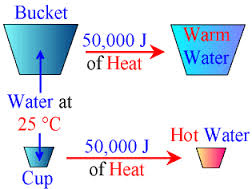# Difference between Temperature and Heat

##### Key Difference: Temperature is the measurement of the average kinetic energy of the particles of an object. It measures the degree of hotness or coldness. On the other hand, heat is basically the energy transfer between objects due to the temperature variation. It flows from the high temperature location to low temperature location.

In day-to-day life, one considers that temperature and heat are the same. However, they are different from each other but still related to each other. To understand the association of heat and temperature, one must be familiar with the kinetic energy possessed by an object.It was first observed by Count Rumford that on a drilling a hole in cannon barrel heat was produced. It was due to motion that takes place in a object. Whenever, a force acts on a body, and makes it move, work is being done. This energy of motion is known as kinetic energy.

Temperature is basically the measurement of this average kinetic energy of molecules. It means that temperature of a substance will be lower if the average kinetic energy of the molecules of a substance is small, and similarly for a large average kinetic energy the temperature would by high. It provides the measurement that how warm or cold is the object with respect to some standard. Thermometer is used for the measurement of temperature. Temperature is measured in degree Celsius.Heat is basically the energy which is transferred between objects due to the temperature variation.  Temperature is used as a measurement factor for attributes of warm and cold. Heat is related to the temperature as it always flows from hotter to colder objects. Heat is not energy but  is a measurement of energy transfer. For example, when a hot water bottle is pressed against the body, it transfers the hotness into the body. It is different from temperature, as unlike temperature it is dependent on the mass of the substance. For example, 30 grams of water at 70 degree centigrade will possess more heat energy then 25 grams of water at 70 degree centigrade.

Heat can also be described as the amount of thermal energy contained in an object. The measurement of heat is done in joules or J. A Joule is a Newton times a meter. If this heat is provided to an object it tends to increase the temperature of that matter. Heat can be transferred by three mediums – conduction, radiation and convection.

Therefore, heat and temperature are different from each other. An example of iceberg is suitable to differentiate the two. An iceberg contains more heat due to large number of molecules, still the temperature is very low. An object with the higher temperature tends to transfer heat and an object with the lower temperature  tends to be the one receiving the heat from the transfer.

Comparison between Temperature and Heat:

 Temperature Heat Definition It is the measurement of the average kinetic energy of the particles of an object. Heat is basically the energy transfer between objects due to the temperature variation. It flows from the high temperature location to low temperature location. Measurement Kelvin (K), Celsius (C) or Fahrenheit (F). Done in Joules or J Dependency of mass No Yes Can be measured directly Yes, by using a thermometer Calo­rieme­ter General Symbol T Q SI unit Joule Kelvin Thermal Equilibrium Two bodies cannot be in thermal equilibrium if they are at different Temperature. Two bodies can be in a thermal equilibrium without having equal thermal energy.  Their temperature are equal in case.

Image Courtesy: education-portal.com, gcsescience.com